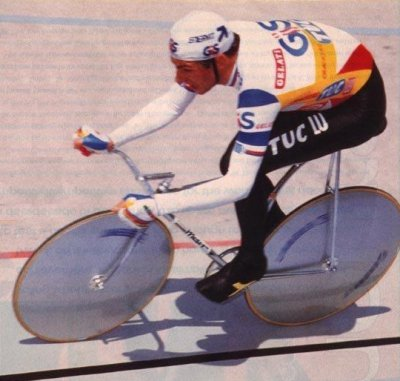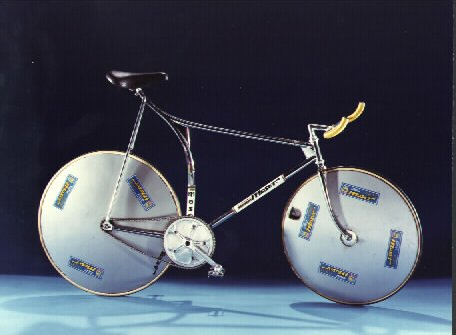Francesco MoserAW197 / Orcus / Logos / Sun conjunction

>>
Francesco Moser (born June 19, 1951 in Palu di Giovo, Province of Trento), nicknamed "Lo sceriffo" (The sheriff)(Logos), is an Italian former professional road bicycle racer. He was one of the dominant riders from the mid-1970s to the early 1980s, and won the 1984 Giro d'Italia, the 1977 world road racing championship and six victories in three of the five classics. He began his professional career in 1973. He had an almost effortless pedal stroke that provided great power. Moser was intimidating (Orcus) on a bicycle but his powerful build (AW197) meant he wasnt a gifted climber.
<<
http://en.wikipedia.org/wiki/Francesco_MoserAssuming noon, 11:00 UT
Using RIYAL 3.13

Astrological Setting (Tropical - Placidus)

RIYAL Tue June 19 1951 UT 11h00m00s Lat46n09 Lon11e09 RADIX

Amycus = 0,02 Tau                 Ceto = 18,33 Ari
Pholus = 1,01 Aqu r                Chariklo = 19,29 Sag r
CY118 = 2,02 Can                  Pelion = 19,37 Can
Cyllarus = 2,26 Sco r              Mercury = 19,52 Gem
Echeclus = 3,28 Can              Mars = 20,06 Gem
Radamantu= 4,16 Can           Thereus = 20,39 Leo
CZ118 = 4,27 Leo                   TL66 = 21,27 Aqu r
Varuna = 4,48 Tau                   Teharonhi= 22,31 Sag r
Asbolus = 6,17 Can                 Pylenor = 23,14 Can
Quaoar = 6,43 Lib r                 (Midheav) = 23,35 Gem
Eris = 8,00 Ari                          Sedna = 24,24 Ari
Uranus = 8,54 Can                 Huya = 24,30 Can
Hylonome = 9,48 Tau               (Ascend) = 24,45 Vir
Crantor = 10,36 Ari                 Saturn = 25,56 Vir
Okyrhoe = 10,43 Ari                Deucalion= 26,24 Leo
Jupiter = 10,57 Ari                 (Moon) = 26,24 Sag
Typhon = 11,06 Ari                   Logos = 26,27 Gem
TD10 = 11,52 Sco r                 Orcus = 26,43 Gem
Venus = 12,37 Leo                 Sun = 27,22 Gem
Node = 13,00 Pis r                   Elatus = 27,34 Aqu r
Ixion = 15,01 Lib r                     Apogee = 28,11 Gem r
Nessus = 15,11 Tau                  Bienor = 29,32 Tau
Neptune = 16,47 Lib r            Chiron = 29,56 Sag r
Pluto = 17,59 Leo                   Chaos = 29,57 Pis
_______________________________________________

Focused Minor Planets

AW197 = 26Ge12
Logos = 26Ge27
Orcus = 26Ge43
Sun = 27Ge22

Saturn = 25Vi56                 Square

Venus = 12Le37                 Semisquare

Deucalion= 26Le24            Sextile
Sedna = 24Ar24

Elatus = 27Aq34 r                Trine

TX300 = 25Cp30 r               Quincunx
_______________________

UQ513 = 19Aq52 r

Mercury = 19Ge52               Trine
Mars = 20Ge06

Ceto = 18Ar33                       Sextile
_______________________

TY364 = 12Aq15 r
RR43 = 12Aq27 r
Venus = 12Le37

KX14 = 12Vi59                   Quincunx

MW12 = 13Li29 r                 Trine
_______________________

Typhon = 11Ar06
Jupiter = 10Ar57
Okyrhoe = 10Ar43
Crantor = 10Ar36

XR190 = 10Ta53                 Semisextile
_______________________

Eris = 8Ar00

OR10 = 8Aq20 r                  Sextile

Uranus = 8Ca54                Square
_______________________

Ixion = 15Li01 r
Neptune = 16Li47 r
_______________________

VQ94 = 15Ar30
Neptune = 16Li47 r

Pluto = 17Le59                   Trine
OM67 = 16Sa14 r
________________________________________________________

Posted to Centaurs (YahooGroups) on July  03, 2010

_________________________________________________________________
_________________________________________________________________